MCAT Physical : Phase Changes

Example Questions

← Previous 1 3 4 5

Example Question #6 : Physical Chemistry

A cubical block has a mass of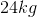and a density of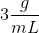. Which of the following values most closely approximates the height of the block?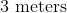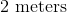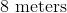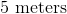Explanation:

To solve this question, you need to know the definition of density: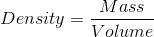The question gives us the mass of the block and the density of the block. We can use these values to solve for the volume of the block.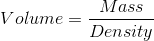Density has to be converted from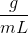to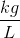(SI units). Recall that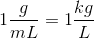; therefore,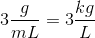.

Solving for volume of the block gives us: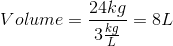A liter is defined as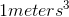; therefore,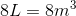.

Remember that the volume of a cube is calculated as follows: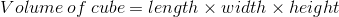Since the cube has the same length, width, and height, the equation above can be rewritten in terms of height as follows: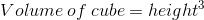This means that the height of the cube can be calculated by taking the cube root of volume: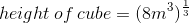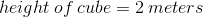The length, the width, and the height of this cubical block is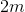.

Example Question #7 : Physical Chemistry

Which of the following is true regarding the density of solids?

Solids usually have greater density than liquids because they have larger volumes

Density of a solid can be calculated by dividing the weight of the solid by the volume of the solid

A sample of a substance in its solid phase will have the same mass as the same sample in its liquid phase

Solid water has a higher density than liquid water

A sample of a substance in its solid phase will have the same mass as the same sample in its liquid phase

Explanation:

Density is defined as mass divided by volume. Recall that the solid phase is usually characterized by tightly packed molecules. As a result, solids usually take up less volume than liquids and, consequently, have greater densities than liquids.

The most common exception to this rule is water. Liquid water has a density of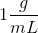, whereas solid water (ice) has a density of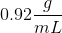.

Density of any substance (solid or liquid) is calculated as follows: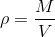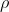is density of the substance,is mass, andis volume. The density of a substance does not depend on the weight of the object.

Changing a substance from its solid phase to its liquid phase only alters volume; it does not change the mass. Remember that the mass of a substance depends on the amount of molecules. Changing from solid to liquid will increase the spacing between the molecules (volume), but it will not change the amount of molecules (mass); therefore, the solid phase and the liquid phase of a sample of substance will have the same mass.

Example Question #8 : Physical Chemistry

What will happen to the density of iron if you increase the temperature to its melting point?

The density will decrease because the mass will decrease

The density will decrease because the volume will increase

The density will increase because the mass will increase

The density will increase because the volume will decrease

The density will decrease because the volume will increase

Explanation:

Increasing a substance’s temperature to its melting point will convert the substance from a solid to a liquid. This will increase the volume of the substance because the molecules within the substance will be farther apart from each other; however, the amount of molecules (mass of the substance) will remain unchanged.

Recall the definition of density:Since the volume increases and the mass stays the same, the density of iron will decrease when you change the temperature to its melting point.

Example Question #9 : Physical Chemistry

Consider the following factors:

I. Temperature

II. Pressure

Which of these two factors will alter the density of a substance?

Only II because it changes the mass of the substance

Only I because it changes the volume of the substance

Both I and II because I changes the volume and II changes the mass of the substance

Both I and II because both change the volume of the substance

Both I and II because both change the volume of the substance

Explanation:

Density is defined as follows:To alter density, we need to alter mass and/or volume. Remember that mass can only be altered by removing from or adding to the sample. Temperature and pressure do not change the amount of substance; therefore, they do not alter the mass of the substance.

Changing temperature will alter the distance between the molecules within the substance. Increasing the temperature will increase the distance between the molecules, which will increase the volume taken up by the substance. Similarly, decreasing the temperature will decrease the distance between the molecules, which will decrease the volume taken up by the substance. This means that changing temperature will alter the volume of the substance and, subsequently, the density of the substance.

Applying pressure also changes the distance between the molecules. Increasing the pressure will force the molecules to be closer together, whereas decreasing the pressure will enable the molecules to spread and separate from each other; therefore, pressure also alters density by changing the volume of the substance.

Example Question #10 : Physical Chemistry

Consider a horizontal tube that has a length of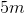and a diameter of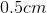. You stretch this tube by applying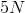of force on each end of the tube. What is happening to the tube when you are applying the force?

The tube is experiencing a stress of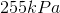and is undergoing tension

The tube is experiencing a stress of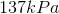and is undergoing compression

The tube is experiencing a stress ofand is undergoing compression

The tube is experiencing a stress ofand is undergoing tension

The tube is experiencing a stress ofand is undergoing tension

Explanation:

To answer this question, you need to solve for the stress experienced by the tube. Stress is defined as: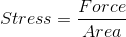Since this is a tube, the area we are concerned with is the circular cross-sectional area of the tube. The question states that the diameter is. This means that the radius (which is half the length of the diameter) is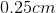. Recall that meters is the SI unit for distance; therefore, we need to convert the radius to meters: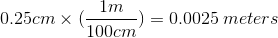This means that the radius of the tube, in meters, is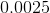. The area of a circle is: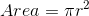Using this formula and our radius, we can find the cross-sectional area: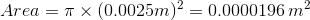The stress experienced by the tube is: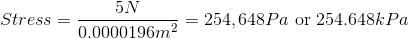The closest answer choice is.

The question states that the tube is being stretched by the force. This means that the tube is undergoing tension because you are pulling the tube and applying a force outwards. If the force was applied in the opposite direction (inwards), then the tube would be undergoing compression (the tube would shorten).

Example Question #1 : Phase Changes

The strain on an object __________ when initial length is increased and __________ when final length is increased.

decreases . . . increases

decreases . . . decreases

increases . . . decreases

increases . . . increases

decreases . . . increases

Explanation:

Strain of an object is defined as: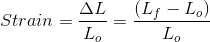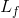is the final length and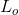is the initial length. The equation implies that increasing the initial length of an object () would decrease the strain. Similarly, the equation also implies that increasing the final length of an object () would increase the strain; therefore, increasing the initial length decreases strain, whereas increasing the final length increases strain.

Example Question #111 : Mcat Physical Sciences

Which of the following will result in the greatest increase in length of a rubber band at its maximum stretch point, as compared to an unaltered control?

Assume the rubber band will experience the same constant force.

Using a rubber band that has half the length

Using a rubber band that has twice the cross-sectional area

Using a rubber band that is twice as elastic

Using a rubber band that is twice as long and is twice as elastic

Using a rubber band that is twice as elastic

Explanation:

To answer this question, we need to know the equation for Young’s modulus,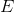: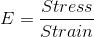Recall the definitions of stress and strain: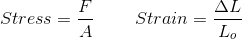Here,is force,is cross-sectional area,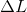is change in length, andis initial length. Plugging these two equations into the definition of Young’s modulus gives us: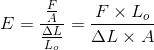Rearranging this equation to solve for change in length gives us: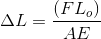Since we want to increasewe look for answer choices that increaseand, or decreaseand. Among the answer choices, the greatest increase occurs when you double the elasticity of the rubber band. Recall that Young’s modulus is a measure of the stiffness of a material; therefore, a more elastic (flexible, or less stiff) material will lower Young’s modulus. Having a material twice as elastic will decreaseby one half and will give the greatest increase in the change in length of the rubber band.

Using half the length () and using twice the cross-sectional area will decrease the change in length. Using a rubber band twice as long, but twice as elastic, will not alter the change in length.

Example Question #112 : Mcat Physical Sciences

Which of the following is false regarding Young’s modulus?

Young’s modulus increases as stress increases

Young’s modulus is defined as the slope of the stress vs. strain plot

Young’s modulus is only valid for certain stresses

Young’s modulus has the same units as stress

Young’s modulus increases as stress increases

Explanation:

Young’s modulus is a constant that is calculated by generating a stress vs. strain plot. The slope of the linear region of the stress vs. strain plot is defined as the Young’s modulus. Recall that the stress vs. strain plot is nonlinear past the elastic limit (stress at which the material starts to plastically deform). This means that we cannot calculate the slope past the elastic limit; Young’s modulus is invalid for stresses beyond the elastic limit.

Young’s modulus is defined as follows: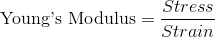To calculate strain, you divide change in length by initial length. Since both change in length and initial length have the same units, the units cancel and strain becomes a dimensionless quantity. This means that the Young’s modulus will have the same units as stress alone.

Young’s modulus is a material property. This means that the Young’s modulus is constant for a given type of material. It does not depend on the stress experienced by the material. Any changes to stress will be compensated for by a change in strain, which will allow Young’s modulus to be constant for the material. Similarly, Young’s modulus does not depend on strain or on the geometrical properties (length and area) of the material. It will only change if you change the type of material being used.

Example Question #1 : Thermodynamics Of Phase Changes

A scientist prepares an experiment to demonstrate the second law of thermodynamics for a chemistry class. In order to conduct the experiment, the scientist brings the class outside in January and gathers a cup of water and a portable stove.

The temperature outside is –10 degrees Celsius. The scientist asks the students to consider the following when answering his questions:

Gibbs Free Energy Formula:

ΔG = ΔH – TΔS

Liquid-Solid Water Phase Change Reaction:

H2O(l) ⇌ H2O(s) + X

The scientist prepares two scenarios.

Scenario 1:

The scientist buries the cup of water outside in the snow, returns to the classroom with his class for one hour, and the class then checks on the cup. They find that the water has frozen in the cup.

Scenario 2:

The scientist then places the frozen cup of water on the stove and starts the gas. The class finds that the water melts quickly.

After the water melts, the scientist asks the students to consider two hypothetical scenarios as a thought experiment.

Scenario 3:

Once the liquid water at the end of scenario 2 melts completely, the scientist turns off the gas and monitors what happens to the water. Despite being in the cold air, the water never freezes.

Scenario 4:

The scientist takes the frozen water from the end of scenario 1, puts it on the active stove, and the water remains frozen.

Which of the following are possible quantities for the “X” present on the right side of the Liquid-Solid Water Phase Change Reaction?

I. Entropy

II. Heat

III. Water Vapor

I, II, and III

I only

I and II

I and III only

II only

II only

Explanation:

As water freezes, it releases heat into the universe to balance the local loss of entropy encountered by ordering atoms into a rigid solid structure. Entropy, in contrast, is not a commodity that can be released by a reaction in the same way as heat. Also, water vapor would not be released on only one side of the equation. Water vapor would exist with in equilibrium with both solid and liquid, but would not appear as an additional product with the solid phase in this reaction.

Example Question #2 : Thermodynamics Of Phase Changes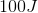of energy is added to a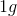sample, which undergoes a phase change from liquid to gas as a result. The temperature remains constant throughout the process. What does thequantity represent?

Specific heat capacity

Specific heat of vaporization

Heat of vaporization

Enthalpy of vaporization